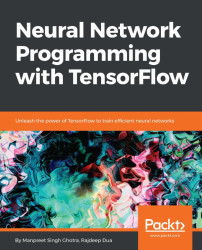•#### Neural Network Programming with Tensorflow#### Overview of this book

If you're aware of the buzz surrounding the terms such as "machine learning," "artificial intelligence," or "deep learning," you might know what neural networks are. Ever wondered how they help in solving complex computational problem efficiently, or how to train efficient neural networks? This book will teach you just that. You will start by getting a quick overview of the popular TensorFlow library and how it is used to train different neural networks. You will get a thorough understanding of the fundamentals and basic math for neural networks and why TensorFlow is a popular choice Then, you will proceed to implement a simple feed forward neural network. Next you will master optimization techniques and algorithms for neural networks using TensorFlow. Further, you will learn to implement some more complex types of neural networks such as convolutional neural networks, recurrent neural networks, and Deep Belief Networks. In the course of the book, you will be working on real-world datasets to get a hands-on understanding of neural network programming. You will also get to train generative models and will learn the applications of autoencoders. By the end of this book, you will have a fair understanding of how you can leverage the power of TensorFlow to train neural networks of varying complexities, without any hassle. While you are learning about various neural network implementations you will learn the underlying mathematics and linear algebra and how they map to the appropriate TensorFlow constructs.
Title PageCreditswww.PacktPub.comCustomer FeedbackPrefaceFree Chapter
Maths for Neural NetworksDeep Feedforward NetworksOptimization for Neural NetworksConvolutional Neural NetworksRecurrent Neural NetworksGenerative ModelsDeep Belief NetworkingAutoencodersResearch in Neural NetworksGetting started with TensorFlow## Avoiding overfitting in neural networks

Let's understand the constituents of overfitting and how to avoid it in neural networks. Nitesh Srivastava, Geoffrey Hinton, et al. published a paper, https://www.cs.toronto.edu/~hinton/absps/JMLRdropout.pdf, in 2014, which shows cases on how to avoid overfitting.

### Problem statement

Deep neural networks contain nonlinear hidden layers, and this makes them expressive models that can learn very complicated relationships between inputs and outputs. However, these complicated relationships will be the result of sampling noise. These complicated relationships might not exist in test data, leading to overfitting. Many techniques and methods have been developed to reduce this noise. These include stopping the training as soon as performance on a validation set starts getting worse, introducing weight penalties such as L1 and L2 regularization, and soft weight sharing (Nowlan and Hinton, 1992).

### Solution

Dropout is a technique that addresses performance issues of...